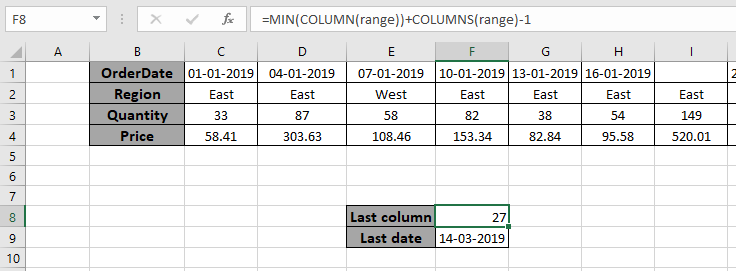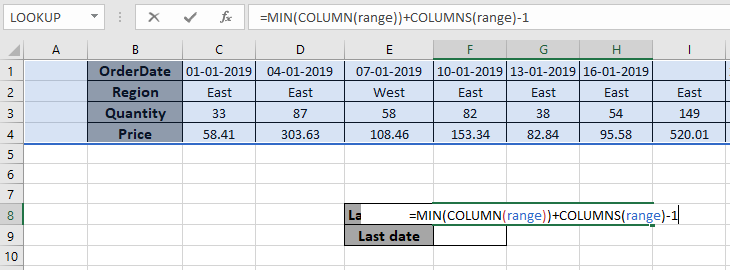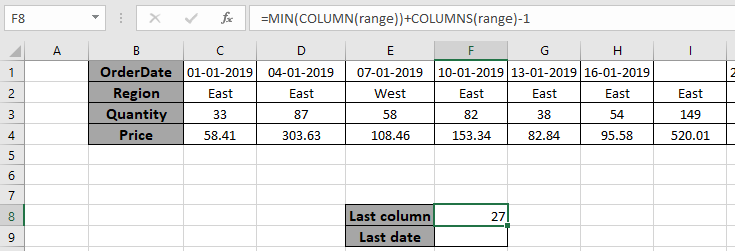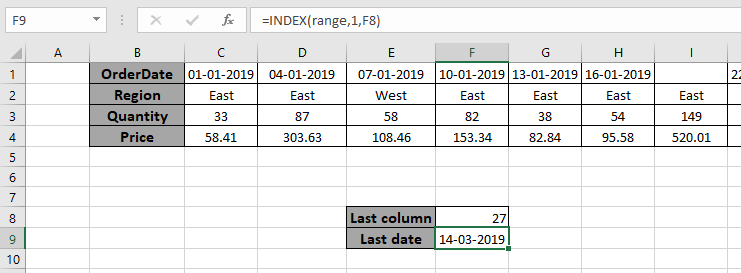How To Find The Last Used Cell in One Column in Microsoft Excel 2010In this article, we will learn How To Find The Last Used Cell in One Column in Microsoft Excel 2010.

Scenario:

In simple words, while working with long unmannerly data, and then if needed to extract the column number of the last cell from range. We can use the below explained formula in Excel and can use the same in formulas to feed the row value. The formula considers all kinds of data types and blank cells in between range arrays.

For this article we will be needing the use the following functions:

Now we will make a formula out of these functions. Here we will give a list of values as data. We need to find the column of the last cell value in the given input data or say the last non-blank cell in the range.

Syntax:

 =MIN(COLUMN(data))+COLUMNS(data)-1

data : list of values having data.

Example:

All of these might be confusing to understand. So, let's test this formula via running it on the example shown below. Here we will perform the formula over values and significance with the range data.

Use the formula:

 =MIN(COLUMN(range))+COLUMNS(range)-1

"range" argument given as named range for the array A1:AA4 in the above formula

Explanation:

• COLUMN function returns a range of values from the first cell column index to the last cell column index.
• COLUMNS function returns the number of columns in the range.
• MIN(COLUMN(range)) returns the lowest count of cells in the column in range.
• =MIN(COLUMN(range))+COLUMNS(range)-1 returns the last column number from the last cell.Here the array to the function is given as the named range. Press Enter to get the last column number as result.As you can see in the above snapshot the column number of the last non blank cell is 27. You can feed the result with other functions to extract different results.

Value from the last non blank cell.

Use the INDEX function to get the value from the last column of data.

Use the formula:

 =INDEX(range,1,F8)As you can see from the above formula you can get the date value from the last column as well.

Here are some observational notes using the above formula.

Notes:

1. The formula returns the last column index as a result.
2. The formula returns a number and can be fed to other functions as number argument.
3. Named range in the formula be used with correct keywords.

Hope this article about How To Find The Last Used Cell in One Column in Microsoft Excel 2010 is explanatory. Find more articles on reference formulas here. If you liked our blogs, share it with your fristarts on Facebook. And also you can follow us on Twitter and Facebook. We would love to hear from you, do let us know how we can improve, complement or innovate our work and make it better for you. Write to us at info@exceltip.com.

Related Articles

How to Retrieve Latest Price in Excel : It is common to update prices in any business and using the latest prices for any purchase or sales is must. To retrieve the latest price from a list in Excel we use the LOOKUP function. The LOOKUP function fetches the latest price.

VLOOKUP function to calculate grade in Excel : To calculate grades IF and IFS are not the only functions that you can use. The VLOOKUP is more efficient and dynamic for such conditional calculations.To calculate grades using VLOOKUP we can use this formula.

17 Things About Excel VLOOKUP : VLOOKUP is most commonly used for retrieving matched values but VLOOKUP can do a lot more than this. Here are 17 things about VLOOKUP that you should know to use effectively.

LOOKUP the First Text from a List in Excel : The VLOOKUP function works fine with wildcard characters. We can use this to extract the first text value from a given list in excel. Here is the generic formula.

LOOKUP date with last value in list : To retrieve the date that contains the last value we use the LOOKUP function. This function checks for the cell that contains the last value in a vector and then uses that reference to return the date.

Popular Articles :

How to use the IF Function in Excel : The IF statement in Excel checks the condition and returns a specific value if the condition is TRUE or returns another specific value if FALSE.

How to use the VLOOKUP Function in Excel : This is one of the most used and popular functions of excel that is used to lookup value from different ranges and sheets.

How to Use SUMIF Function in Excel : This is another dashboard essential function. This helps you sum up values on specific conditions.

How to use the COUNTIF Function in Excel : Count values with conditions using this amazing function. You don't need to filter your data to count specific values. Countif function is essential to prepare your dashboard.

Terms and Conditions of use

The applications/code on this site are distributed as is and without warranties or liability. In no event shall the owner of the copyrights, or the authors of the applications/code be liable for any loss of profit, any problems or any damage resulting from the use or evaluation of the applications/code.